Chemistry Equilibrium and its type

### Topic to be covered

star Introduction.
star Equilibrium in physical process.
star Solid-Liquid Equilibrium.
star Liquid-Vapour Equilibrium.
star Solid – Vapour Equilibrium.
star Equilibrium Involving Dissolution of Solid or Gases in Liquids.
star General Characteristics of Equilibria Involving Physical Processes

### introduction

=> Chemical equilibria are important in numerous biological and environmental processes.

For example, equilibria involving color{red}(O_2) molecules and the protein hemoglobin play a crucial role in the transport and delivery of color{red}(O_2) from our lungs to our muscles. Similar equilibria involving color{red}(CO) molecules and hemoglobin account for the toxicity of color{red}(CO).

=> When a liquid evaporates in a closed container, molecules with relatively higher kinetic energy escape the liquid surface into the vapour phase and number of liquid molecules from the vapour phase strike the liquid surface and are retained in the liquid phase.

=> It gives rise to a constant vapour pressure because of an equilibrium in which the number of molecules leaving the liquid equals the number returning to liquid from the vapour.

=> We say that the system has reached equilibrium state at this stage. However, this is not static equilibrium and there is a lot of activity at the boundary between the liquid and the vapour. Thus, at equilibrium, the rate of evaporation is equal to the rate of condensation. It may be represented by

color{red}(H_2O (l) ⇌ H_2O text{(vap)})

color{red}("The double half arrows indicate that the processes in")
color{red}(" both the directions are going on simultaneously. ") color{red}("The mixture of reactants and products in") color{red}(" the equilibrium state is called an equilibrium mixture").

=> Equilibrium can be established for both physical processes and chemical reactions.

=> When the reactants in a closed vessel at a particular temperature react to give products, the concentrations of the reactants keep on decreasing, while those of products keep on increasing for some time after which there is no change in the concentrations of either of the reactants or products. This stage of the system is the dynamic equilibrium and the rates of the forward and reverse reactions become equal (there is no change in the concentrations of various species in the reaction mixture).

=>Based on the extent to which the reactions proceed to reach the state of chemical equilibrium, these may be classified in three groups.

(i) The reactions that proceed nearly to completion and only negligible concentrations of the reactants are left. In some cases, it may not be even possible to detect these experimentally.

(ii) The reactions in which only small amounts of products are formed and most of the reactants remain unchanged at equilibrium stage.

(iii) The reactions in which the concentrations of the reactants and products are comparable, when the system is in equilibrium.

color{purple}(✓✓)color{purple} " DEFINITION ALERT"
Equilibrium represents the state of a process in which the properties like temperature, pressure, concentration of the system do not change with the passage of time.

### EQUILIBRIUM IN PHYSICAL PROCESSES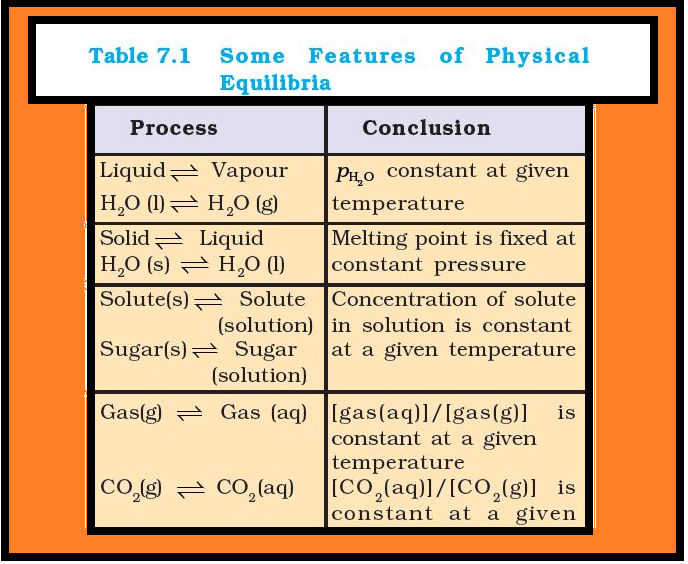The characteristics of system at equilibrium are better understood if we examine some physical processes. The most familiar examples are phase transformation processes, e.g.,

solid ⇌ liquid
liquid ⇌ gas
solid ⇌ gas

### Solid-Liquid Equilibrium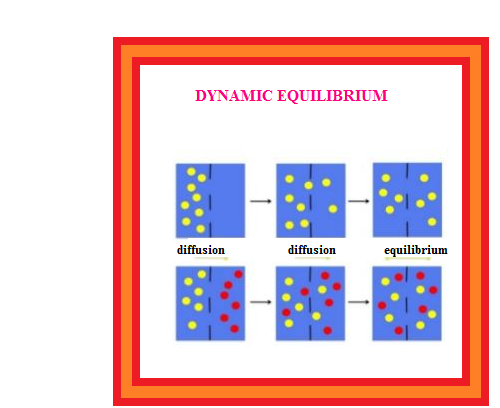=> Ice and water kept in a perfectly insulated thermos flask (no exchange of heat between its contents and the surroundings) at 273K and the atmospheric pressure are in equilibrium state and we observe that the mass of ice and water do not change with time and the temperature remains constant. However, the equilibrium is not static. The intense activity can be noticed at the boundary between ice and water. Molecules from the liquid water collide against ice and adhere to it and some molecules of ice escape into liquid phase.

=> There is no change of mass of ice and water, as the rates of transfer of molecules from ice into water and of reverse transfer from water into ice are equal at atmospheric pressure and 273 K.

When the system is in dynamic equilibrium and we can infer the following:

(i) Both the opposing processes occur simultaneously.
(ii) Both the processes occur at the same rate so that the amount of ice and water remains constant.

### Liquid-Vapour Equilibrium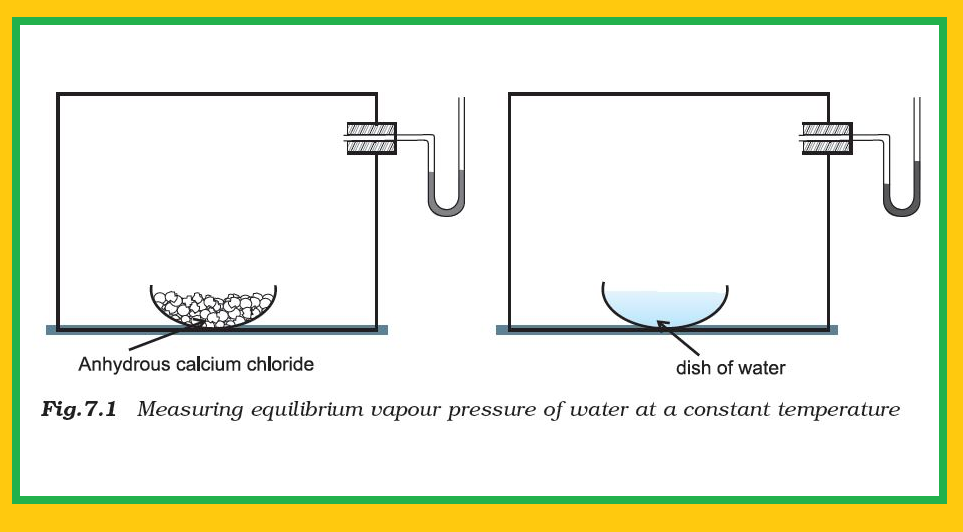=> This equilibrium can be better understood if we consider the example of a transparent box carrying a U-tube with mercury (manometer). Drying agent like anhydrous calcium chloride (or phosphorus penta-oxide) is placed for a few hours in the box. After removing the drying agent by tilting the box on one side, a watch glass (or petri dish) containing water is quickly placed inside the box.

=> It will be observed that the mercury level in the right limb of the manometer slowly increases and finally attains a constant value, that is, the pressure inside the box increases and reaches a constant value. Also the volume of water in the watch glass decreases (Fig. 7.1).
=> Initially there was no water vapour (or very less) inside the box. As water evaporated, the pressure in the box increased due to addition of water molecules into the gaseous phase inside the box. The rate of evaporation is constant. However, the rate of increase in pressure decreases with time due to condensation of vapour into water. Finally it leads to an equilibrium condition when there is no net evaporation. This implies that the number of water molecules from the gaseous state into the liquid state also increases till the equilibrium is attained i.e.,

rate of evaporation= rate of condensation

color{red}(H_2O(l) ⇌ H_2O) text{(vap)})

=> At equilibrium the pressure exerted by the water molecules at a given temperature remains constant and is called the equilibrium vapour pressure of water (or just vapour pressure of water); vapour pressure of water increases with temperature. If the above experiment is repeated with methyl alcohol, acetone and ether, it is observed that different liquids have different equilibrium vapour pressures at the same temperature, and the liquid which has a higher vapour pressure is more volatile and has a lower boiling point

=> If we expose three watch glasses containing separately 1mL each of acetone, ethyl alcohol, and water to atmosphere and repeat the experiment with different volumes of the liquids in a warmer room, it is observed that in all such cases the liquid eventually disappears and the time taken for complete evaporation depends on (i) the nature of the liquid, (ii) the amount of the liquid and (iii) the temperature. When the watch glass is open to the atmosphere, the rate of evaporation remains constant but the molecules are dispersed into large volume of the room. As a consequence the rate of condensation from vapour to liquid state is much less than the rate of evaporation. These are open systems and it is not possible to reach equilibrium in an open system.

Water and water vapour are in equilibrium position at atmospheric pressure (1.013 bar) and at 100°C in a closed vessel. The boiling point of water is 100°C at 1.013 bar pressure. For any pure liquid at one atmospheric pressure (1.013 bar), the temperature at which the liquid and vapours are at equilibrium is called normal boiling point of the liquid. Boiling point of the liquid depends on the atmospheric pressure. It depends on the altitude of the place; at high altitude the boiling point decreases.

### Solid – Vapour Equilibrium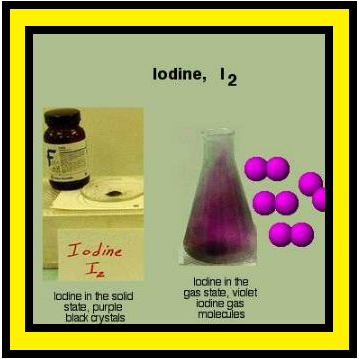=> Let us now consider the systems where solids sublime to vapour phase. If we place solid iodine in a closed vessel, after sometime the vessel gets filled up with violet vapour and the intensity of colour increases with time. After certain time the intensity of colour becomes constant and at this stage equilibrium is attained. Hence solid iodine sublimes to give iodine vapour and the iodine vapour condenses to give solid iodine. The equilibrium can be represented as,

color{red}(I_2text{(solid)} ⇌ I_2) text{(vapour)})

Other examples showing this kind of equilibrium are,

Camphor (solid) ⇌ Camphor (vapour)

color{red}(NH_4Cl text{(solid)} ⇌ NH_4Cl text{(vapour)})

### Equilibrium Involving Dissolution of Solid or Gases in Liquids

•Solids in liquids : When we make a thick sugar syrup solution by dissolving sugar at a higher temperature, sugar crystals separate out if we cool the syrup to the room temperature (a saturated solution). The concentration of the solute in a saturated solution depends upon the temperature. In a saturated solution, a dynamic equilibrium exits between the solute molecules in the solid state and in the solution:

Sugar (solution) ⇌ Sugar (solid), and the rate of dissolution of sugar = rate of crystallisation of sugar.

Equality of the two rates and dynamic nature of equilibrium has been confirmed with the help of radioactive sugar. If we drop some radioactive sugar into saturated solution of non-radioactive sugar, then after some time radioactivity is observed both in the solution and in the solid sugar. Initially there were no radioactive sugar molecules in the solution but due to dynamic nature of equilibrium, there is exchange between the radioactive and non-radioactive sugar molecules between the two phases. The ratio of the radioactive to nonradioactive molecules in the solution increases till it attains a constant value.

•Gases in liquids : When a soda water bottle is opened, some of the carbon dioxide gas dissolved in it fizzes out rapidly. The phenomenon arises due to difference in solubility of carbon dioxide at different pressures. There is equilibrium between the molecules in the gaseous state and the molecules dissolved in the liquid under pressure i.e.,

color{red}(CO_2 text{(gas)} ⇌ CO_2 text{(in solution)})
This equilibrium is governed by Henry’s law, which states that the mass of a gas dissolved in a given mass of a solvent at any temperature is proportional to the pressure of the gas above the solvent. This amount decreases with increase of temperature.

color{purple}♣ color{Violet} " Just for Curious"
The soda water bottle is sealed under pressure of gas when its solubility in water is high. As soon as the bottle is opened, some of the dissolved carbon dioxide gas escapes to reach a new equilibrium condition required for the lower pressure, namely its partial pressure in the atmosphere. This is how the soda water in bottle when left open to the air for some time, turns ‘flat’. It can be generalised that:

(i) For solid ⇌ liquid equilibrium, there is only one temperature (melting point) at 1 atm (1.013 bar) at which the two phases can coexist. If there is no exchange of heat with the surroundings, the mass of the two phases remains constant.

(ii) For liquid ⇌ vapour equilibrium, the vapour pressure is constant at a given temperature.

(iii) For dissolution of solids in liquids, the solubility is constant at a given temperature.

(iv) For dissolution of gases in liquids, the concentration of a gas in liquid is proportional to the pressure (concentration) of the gas over the liquid. These observations are summarised in Table 7.1

### General Characteristics of Equilibria Involving Physical Processes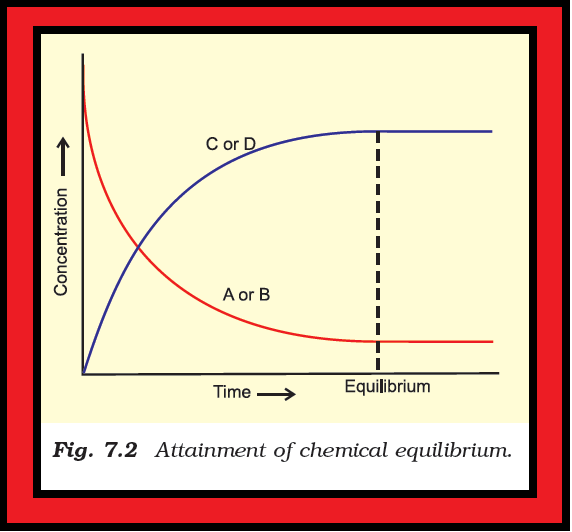For the physical processes discussed above, following characteristics are common to the system at equilibrium:

(i) Equilibrium is possible only in a closed system at a given temperature.

(ii) Both the opposing processes occur at the same rate and there is a dynamic but stable condition.

(iii) All measurable properties of the system remain constant.

(iv) When equilibrium is attained for a physical process, it is characterised by constant value of one of its parameters at a given temperature. Table 7.1 lists such quantities.

(v) The magnitude of such quantities at any stage indicates the extent to which the physical process has proceeded before reaching equilibrium.